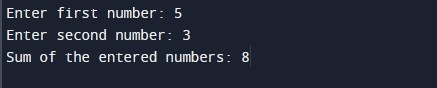C program to add two numbers using function

In this tutorial you will learn about the C program to add two numbers using function and its application with practical example.

In this tutorial, we will learn to create a program to add two integer numbers using function in C programming.

Prerequisites

Before starting with this tutorial we assume that you are best aware of the following C programming topics:

• C Data Types
• C Variables
• C Input Output
• C Operators
• C Function

In this program we will add two integer numbers entered by the user. We would first declared and initialized the required variables. Next, we would prompt user to input two integer numbers. Later in the program we will add the numbers using a user defined function and display the sum of the numbers.

Output:-In the above program, we have first declared and initialized a set variables required in the program.

• num1 = it holds the first integer number value
• num2 = it holds the second integer number value
• num3 = it holds the return value of the sum function

In the next statement user will be prompted to enter the two integer number values which will be assigned to variable ‘num1’ and ‘num2’ respectively. Next, we have called sum function and assigned its return value to ‘num3’. Now, we will be displaying the sum of two integer numbers using printf statement. Later in the last section we have defined a user defined function called sum(), which means to accept two integer numbers and return sum of the numbers passed as parameters.

In this tutorial we have learn about the C program to add two numbers using function and its application with practical example. I hope you will like this tutorial.